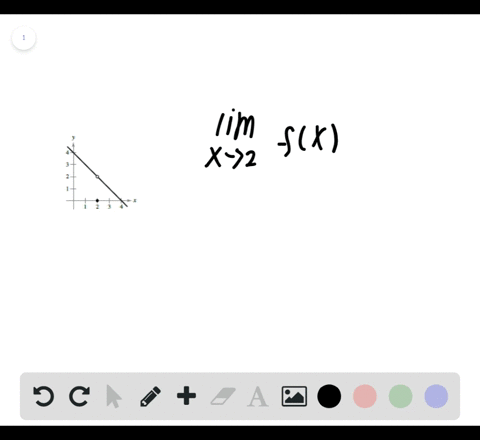Enroll in one of our FREE online STEM bootcamps. Join today and start acing your classes!View Bootcamps00:29
Problem 22

# In Exercises21-28, use the graph to find the limit (if it exists).If the limit does not exist, explain why.$\lim _{x \rightarrow 0} \sec x$

## Discussion

You must be signed in to discuss.

## Video Transcript

and this problem were asked to find the women is except torches zero of seeking of X, and we're given the graph of the function seeking of X to find this limit. So we know the limit exists if the left and right now, let's agree at the given X value that were approaching. So let's study the left hand limits and the white helmets for X equal. Sarah. So if we study the graph from the left hand side of zero, you can see that the Catholics values are getting closer and closer. Two This value right here, which is labelled by the red top. And if we look at it the right hand limits or the behavior of function from the right hand side, we see that the graph is also approaching this. Why value here based on the graph they given, You know that this why values equal to one so we can say. But since the left hand limit is he all to one and the right hand limit is also equal to one. The limit as and Kes approaches zero is in fact want one. In other words, this is an example of one the limit as X approaches see his equal two after seat